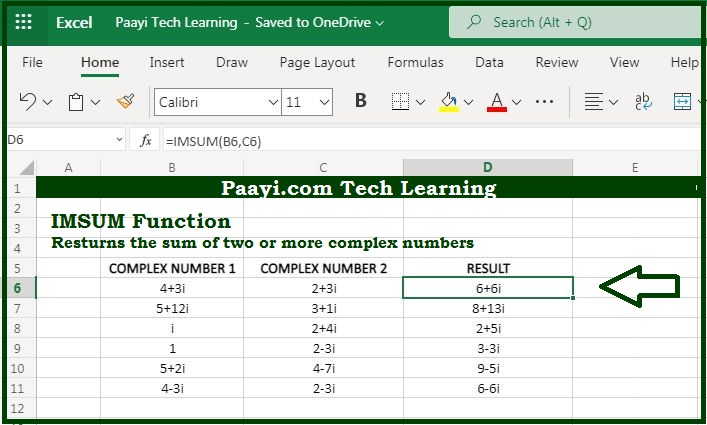# Learn How to Use Microsoft Excel IMSUM Function

Written by | 0 Comments | 528 Views

In this article, you will learn how to use the Microsoft Excel IMSUM function and its prime function in Microsoft Excel. You will also get to know the Microsoft Excel IMSUM function return value and syntax with the help of some examples.

Microsoft Excel IMSUM Function

The prime function of the Microsoft Excel IMSUM function is to get the sum of the two complex numbers. So, with the help of the IMSUM function, you can able to return the sum of the two complex numbers. The complex number should be in the form of  "x + yi" or "x + yj". You can also use the COMPLEX function to create complex numbers from real and imaginary parts.

Return Value of IMSUM Function

The return value will be the sum of the two complex numbers.

Syntax of IMSUM Function

=IMSUM(inumber1, [inumber2], ...)

Where the arguments:

inumber1: It is the complex number 1.

inumber2: It is the complex number 2 (optional).

## How to Use Microsoft Excel IMSUM Function?As we know the IMSUM function you can able to return the sum of the two complex numbers. The complex number is input in the form text as,  "x + yi" or "x + yj".